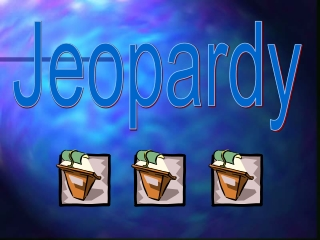DownloadDownload PresentationJeopardy

# Jeopardy

Download Presentation## Jeopardy

- - - - - - - - - - - - - - - - - - - - - - - - - - - E N D - - - - - - - - - - - - - - - - - - - - - - - - - - -
##### Presentation Transcript

1. Jeopardy

2. Multiply and Divide Rational Expressions Adding, Subtracting and Solving Rational Functions Reciprocal Functions Variation 100 100 100 100 100 200 200 200 200 200 Jeopardy 300 300 300 300 300 Final Jeopardy

3. Multiplication for 100

4. Multiply and Divide for 200

5. Multiply and Divide for 300

6. Rational Functions for 100 Find the roots of:

7. Rational Functions for 200 Find the vertical asymptote and holes for:

8. Rational Functions for 300 Find the horizontal asymptote for

9. State the shifts of the graph below from the parent function: Reciprocal Functions 100

10. Reciprocal Functions 200 Name the vertical asymptote of the graph of g(x) and state its domain:

11. What is the horizontal asymptote of: Reciprocal Functions 300

12. Gravitational potential energy, PE, is a measure of energy. PE varies directly with an object’s mass m and its height h in meters above the ground. If a skateboarder is on a half pipe 4 m above the ground and he weighs 58 kg and has a potential energy of 2273.56 joules, what is the gravitational potential energy of a 65 kg skateboard on the same half-pipe? Variation 100

13. The number of bags of grass seed, n, needed to reseed a yard varies directly with the area, a, to be seeded and inversely with the weight, w, of a bag of seed. If it takes two 3 lb bags to seed an area of 3600 ft2, how many 3 lb bags will seed 9000 ft2? Variation 200

14. The number of bags of mulch you need to cover a planting area varies jointly with the area to be mulched a in square feet and the depth of the mulch d in feet. If you need 10 bags to mulch 120 ft2 to a depth of 3in, how many bags do you need to mulch 200 ft2 to a depth of 4 in? Variation 300

15. Adding, Subtracting, and Solving for 100

16. Adding, Subtracting, and Solving for 100

17. Adding, Subtracting, and Solving for 100

18. Final Jeopardy